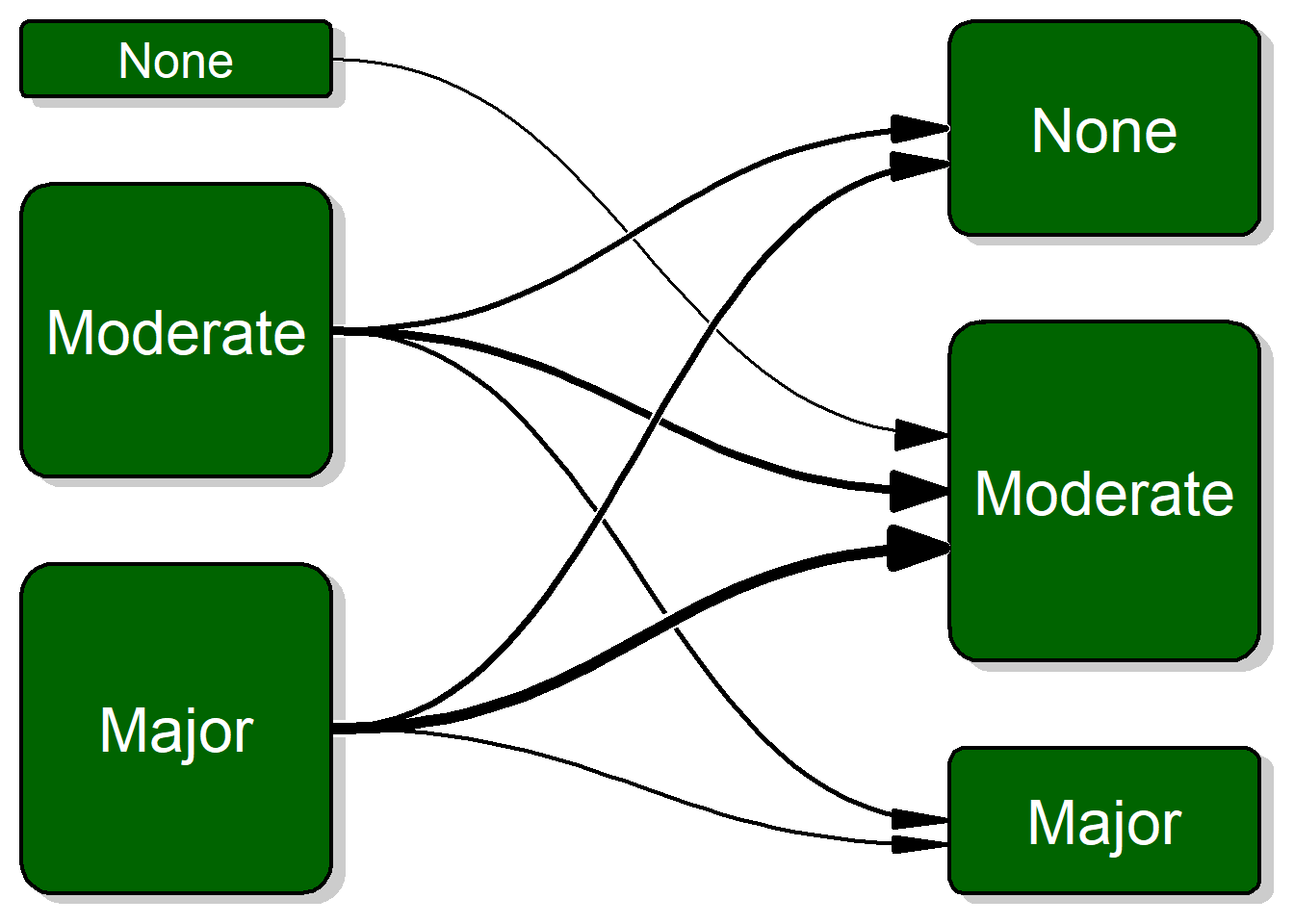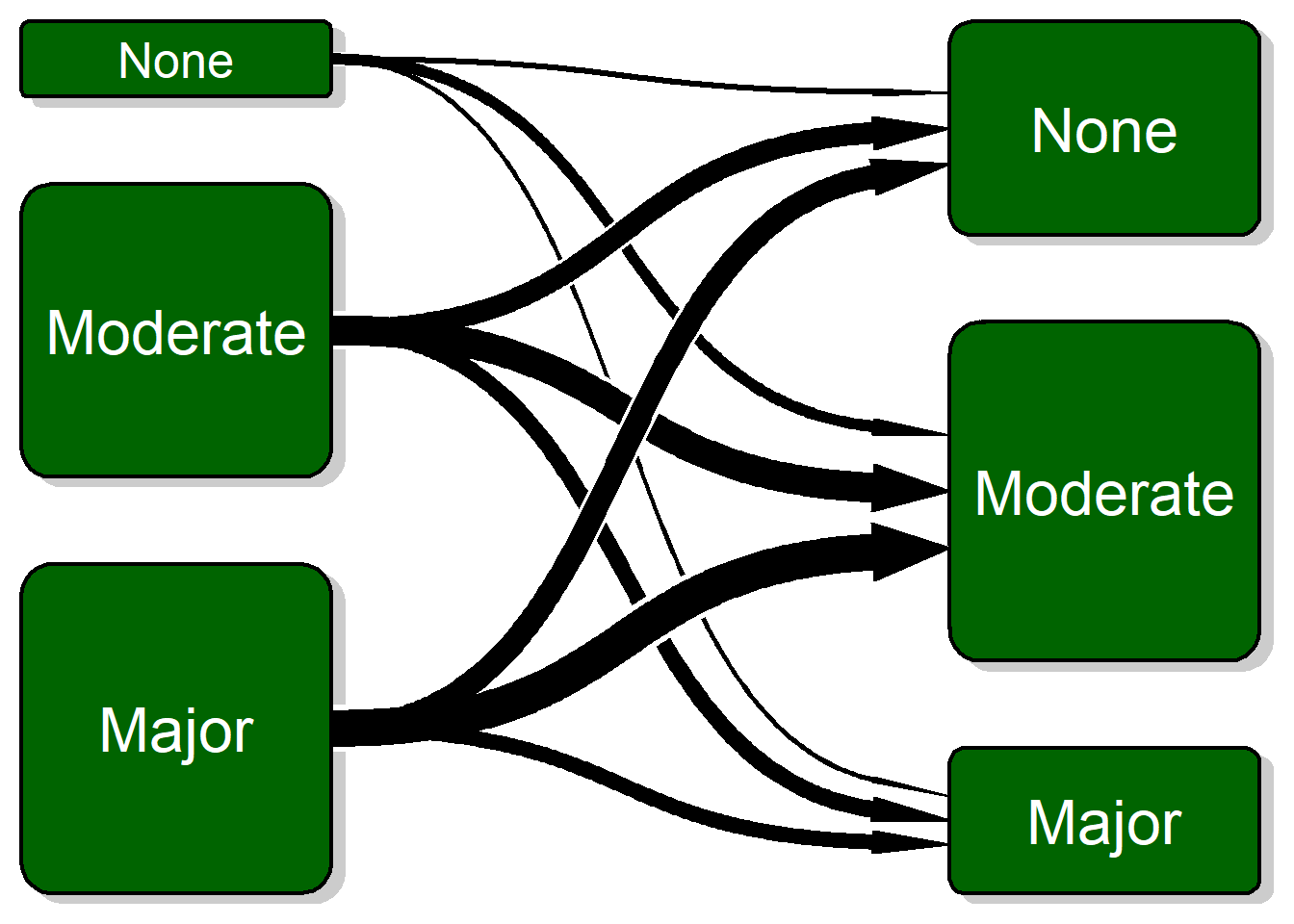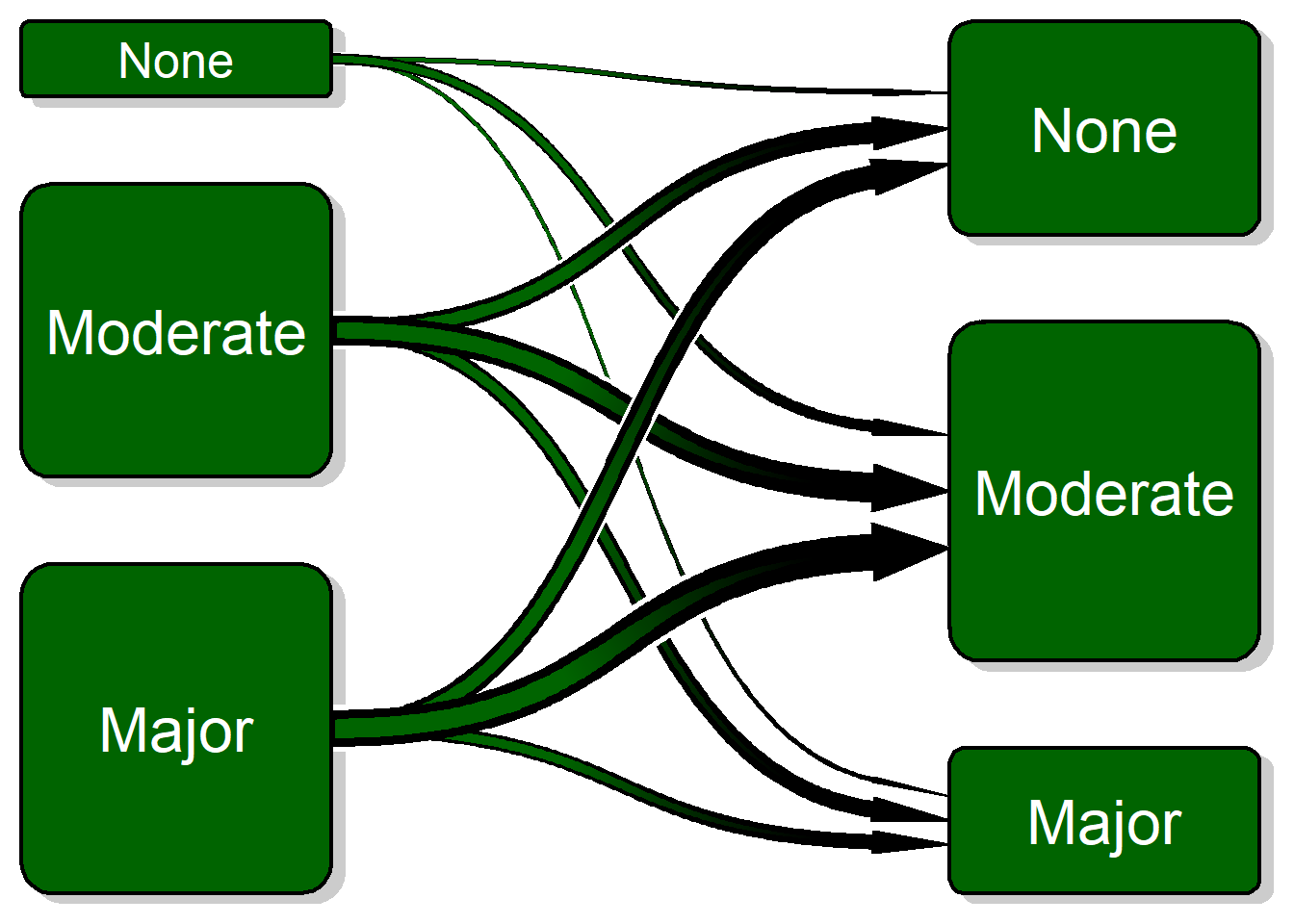## Transition plot

It’s closely related to the `plotMat` for plotting networks but aimed at less complex relations with only a one-way relation between two groups of states. Wait till you see this - it is something you can likely use all the time in your job!

## Simulate some data

We start by creating some sample data/

``````before <- sample(1:3, replace = TRUE, size = 500, prob = c(0.1, 0.4, 0.5))
after <- sample(1:3, replace = TRUE, size = 500, prob = c(0.3, 0.5, 0.2))
before <- factor(before, labels = c("None", "Moderate", "Major"))
after <- factor(after, labels = c("None", "Moderate", "Major"))
# Create the transition matrix
transition_matrix <- table(before, after)
# Create a table with the transitions
htmlTable(transition_matrix, title = "Transitions", ctable = TRUE)``````
None Moderate Major
None 13 32 9
Moderate 66 94 50
Major 74 117 45

## Basic Example

This is simple to understand but understanding the flow can be challenging and time-consuming. Visualizing it using the transition plot gives a quick and more intuitive understanding:

``transitionPlot(transition_matrix)``Here you can see the thick lines representing the transition from that particular group into the next. So far I’ve used the graph for the same measure on left and right side but I guess your imagination sets the limit. It can just as well be two or more treatment arms with some discrete outcomes.

### Basic Improvement - Arrows

Change the arrow type to my alternative arrow we get a nicer plot:

``transitionPlot(transition_matrix, overlap_add_width = 1.3, type_of_arrow = "simple")``### Basic Improvement - Arrows with Gradient

Added a white background to each arrow in order to visually separate the arrows (the overlay order can be customized with the overlap order parameter). To further enhance the transition add the option of a color gradient. To do this you need to specify arrow_type = “gradient”:

``transitionPlot(transition_matrix, overlap_add_width = 1.3, type_of_arrow = "gradient")````````transitionPlot(transition_matrix, txt_start_clr = "black", txt_end_clr = "black",